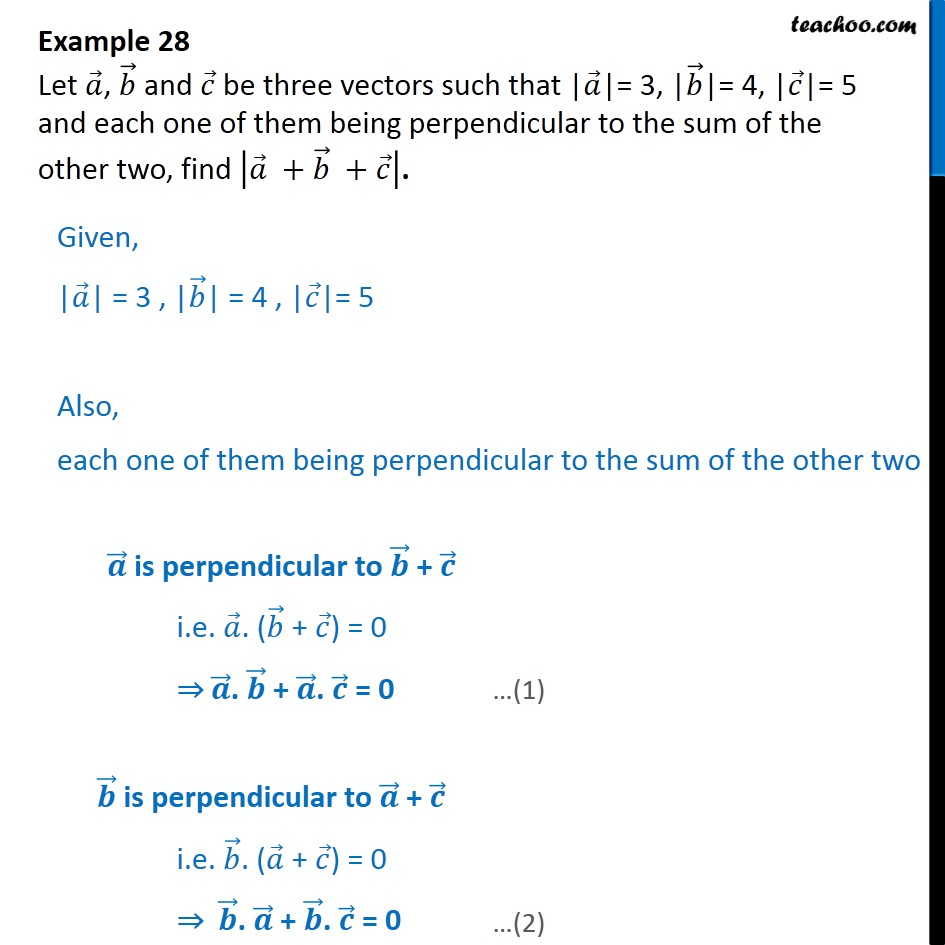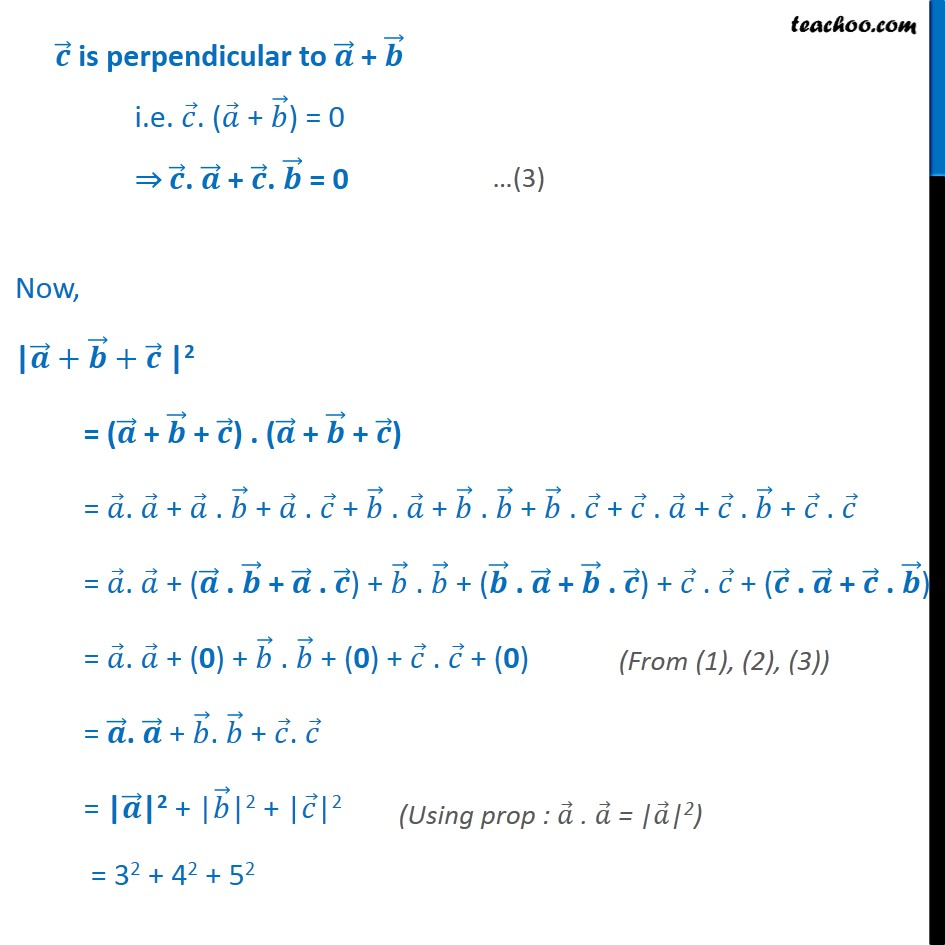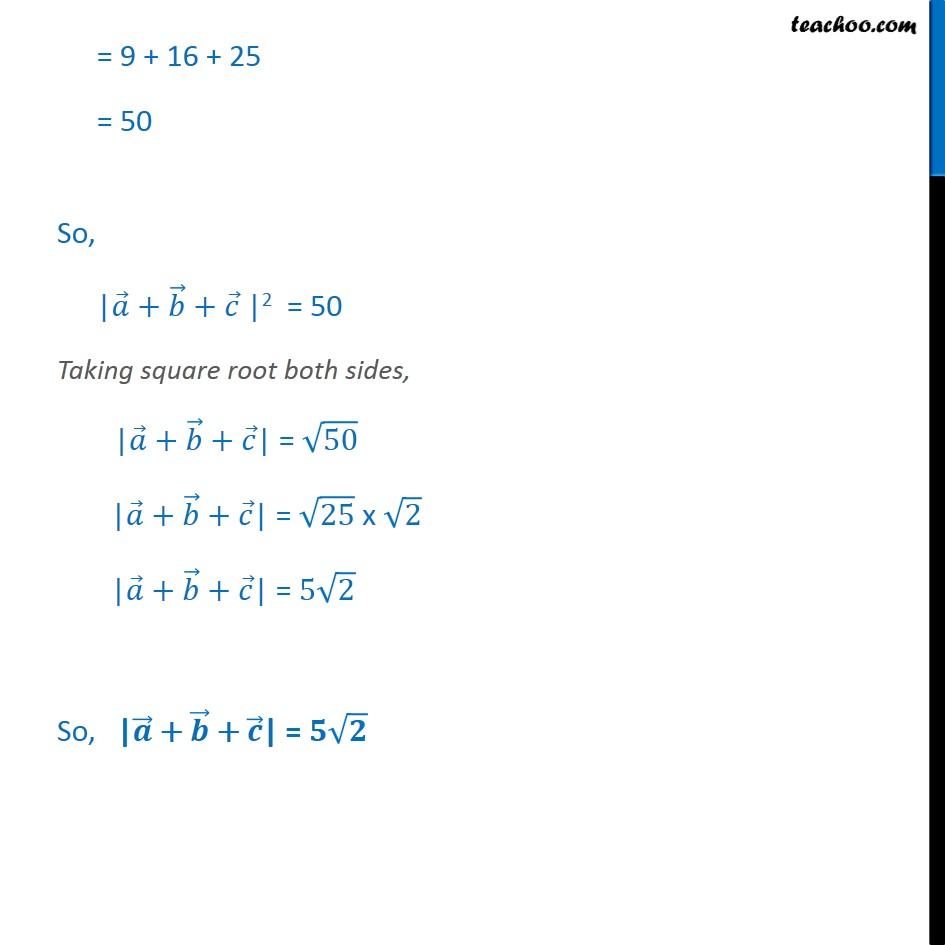1. Class 12
2. Important Question for exams Class 12
3. Chapter 10 Class 12 Vector Algebra

Transcript

Example 28 Let 𝑎﷯, 𝑏﷯ and 𝑐﷯ be three vectors such that | 𝑎﷯|= 3, | 𝑏﷯|= 4, | 𝑐﷯|= 5 and each one of them being perpendicular to the sum of the other two, find 𝑎﷯ + 𝑏﷯ + 𝑐﷯﷯. Given, | 𝑎﷯| = 3 , | 𝑏﷯| = 4 , | 𝑐﷯|= 5 Also, each one of them being perpendicular to the sum of the other two 𝒂﷯ is perpendicular to 𝒃﷯ + 𝒄﷯ i.e. 𝑎﷯. ( 𝑏﷯ + 𝑐﷯) = 0 ⇒ 𝒂﷯. 𝒃﷯ + 𝒂﷯. 𝒄﷯ = 0 𝒃﷯ is perpendicular to 𝒂﷯ + 𝒄﷯ i.e. 𝑏﷯. ( 𝑎﷯ + 𝑐﷯) = 0 ⇒ 𝒃﷯. 𝒂﷯ + 𝒃﷯. 𝒄﷯ = 0 𝒄﷯ is perpendicular to 𝒂﷯ + 𝒃﷯ i.e. 𝑐﷯. ( 𝑎﷯ + 𝑏﷯) = 0 ⇒ 𝒄﷯. 𝒂﷯ + 𝒄﷯. 𝒃﷯ = 0 Now, | 𝒂﷯+ 𝒃﷯+ 𝒄﷯ |2 = ( 𝒂﷯ + 𝒃﷯ + 𝒄﷯) . ( 𝒂﷯ + 𝒃﷯ + 𝒄﷯) = 𝑎﷯. 𝑎﷯ + 𝑎﷯ . 𝑏﷯ + 𝑎﷯ . 𝑐﷯ + 𝑏﷯ . 𝑎﷯ + 𝑏﷯ . 𝑏﷯ + 𝑏﷯ . 𝑐﷯ + 𝑐﷯ . 𝑎﷯ + 𝑐﷯ . 𝑏﷯ + 𝑐﷯ . 𝑐﷯ = 𝑎﷯. 𝑎﷯ + ( 𝒂﷯ . 𝒃﷯ + 𝒂﷯ . 𝒄﷯) + 𝑏﷯ . 𝑏﷯ + ( 𝒃﷯ . 𝒂﷯ + 𝒃﷯ . 𝒄﷯) + 𝑐﷯ . 𝑐﷯ + ( 𝒄﷯ . 𝒂﷯ + 𝒄﷯ . 𝒃﷯) = 𝑎﷯. 𝑎﷯ + (0) + 𝑏﷯ . 𝑏﷯ + (0) + 𝑐﷯ . 𝑐﷯ + (0) = 𝒂﷯. 𝒂﷯ + 𝑏﷯. 𝑏﷯ + 𝑐﷯. 𝑐﷯ = | 𝒂﷯|2 + | 𝑏﷯|2 + | 𝑐﷯|2 = 32 + 42 + 52 = 9 + 16 + 25 = 50 So, | 𝑎﷯+ 𝑏﷯+ 𝑐﷯ |2 = 50 Taking square root both sides, | 𝑎﷯ + 𝑏﷯ + 𝑐﷯| = ﷮50﷯ | 𝑎﷯ + 𝑏﷯ + 𝑐﷯| = ﷮25﷯ x ﷮2﷯ | 𝑎﷯ + 𝑏﷯ + 𝑐﷯| = 5 ﷮2﷯ So, | 𝒂﷯ + 𝒃﷯ + 𝒄﷯| = 𝟓 ﷮𝟐﷯

Chapter 10 Class 12 Vector Algebra

Class 12
Important Question for exams Class 12